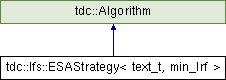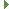tudocomp – The TU Dortmund Compression Framework
tdc::lfs::ESAStrategy< text_t, min_lrf > Class Template Reference

`#include <ESAStrategy.hpp>`

Inheritance diagram for tdc::lfs::ESAStrategy< text_t, min_lrf >:## Public Member Functions

void compute_rules (io::InputView &input, rules &dictionary, non_terminal_symbols &nts_symbols)Public Member Functions inherited from tdc::Algorithm
virtual ~Algorithm ()=default

Algorithm (Algorithm const &)=default

Algorithm (Algorithm &&)=default

Algorithmoperator= (Algorithm const &)=default

Algorithmoperator= (Algorithm &&)=default

Algorithm (Env &&env)
Instantiates an algorithm in the specified environment. More...

Envenv ()

const Envenv () const

## Static Public Member Functions

static Meta meta ()

## Detailed Description

### template<typename text_t = TextDS<>, uint min_lrf = 2> class tdc::lfs::ESAStrategy< text_t, min_lrf >

Definition at line 16 of file ESAStrategy.hpp.

## ◆ compute_rules()

template<typename text_t = TextDS<>, uint min_lrf = 2>
 void tdc::lfs::ESAStrategy< text_t, min_lrf >::compute_rules ( io::InputView & input, rules & dictionary, non_terminal_symbols & nts_symbols )
inline

Definition at line 67 of file ESAStrategy.hpp.

## ◆ meta()

template<typename text_t = TextDS<>, uint min_lrf = 2>
 static Meta tdc::lfs::ESAStrategy< text_t, min_lrf >::meta ( )
inlinestatic

Definition at line 56 of file ESAStrategy.hpp.

The documentation for this class was generated from the following file: